Question

# A company has sales of \$65,000 and total costs of \$14,000, including depreciation and interest expenses....

A company has sales of \$65,000 and total costs of \$14,000, including depreciation and interest expenses. The average tax rate is 50%.

Total assets are \$73,000 and total equity is \$36,000.

What is the company's net income?

What is the total debt ratio (including all liabilities)?

 Sales revenue 65000 Less: Cost including dep and interest 14000 Pre tax Income 51000 Less: tax @ 50% 25500 After tax Income 25500 Total assets 73000 Less: Total Equity 36000 Total debts 37000 Total debts ratio = Total debts / Total assets *100 37000/73000* 100 = 50.68%

#### Earn Coins

Coins can be redeemed for fabulous gifts.

Similar Homework Help Questions
• ### Module 2 Assignment ABC Inc. 2020 sales are \$1,000,000. Operating costs (excluding depreciation) are 65% of...

Module 2 Assignment ABC Inc. 2020 sales are \$1,000,000. Operating costs (excluding depreciation) are 65% of sales. Net fixed assets are \$195,000. Depreciation amounted to 12% of net fixed assets. Interest expenses are \$105,000. The tax bill must be calculated using the corporate income tax table in the text, and ABC Inc. paid 8% of net income in dividends. Prepare ABC Inc.’s income statement for 2018: ABC Inc. Income Statement 2020 Sales Operating costs (excluding depreciation) EBITDA Depreciation EBIT Interest...

• ### Intro Long Gone Corp. had total operating expenses of \$84 million last year, including depreciation, and...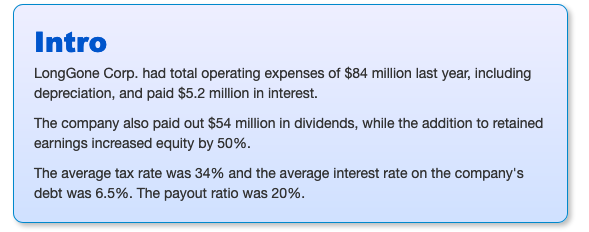Intro Long Gone Corp. had total operating expenses of \$84 million last year, including depreciation, and paid \$5.2 million in interest. The company also paid out \$54 million in dividends, while the addition to retained earnings increased equity by 50%. The average tax rate was 34% and the average interest rate on the company's debt was 6.5%. The payout ratio was 20%. IB Attempt 1/10 for 10 pts. Part 3 What was the equity multiplier? k+ decimals Submit

• ### 524257 374554 10000 5000 29408 1000 Sales Operating Costs Depreciation Expense Interest Expense Tax Expense Cash...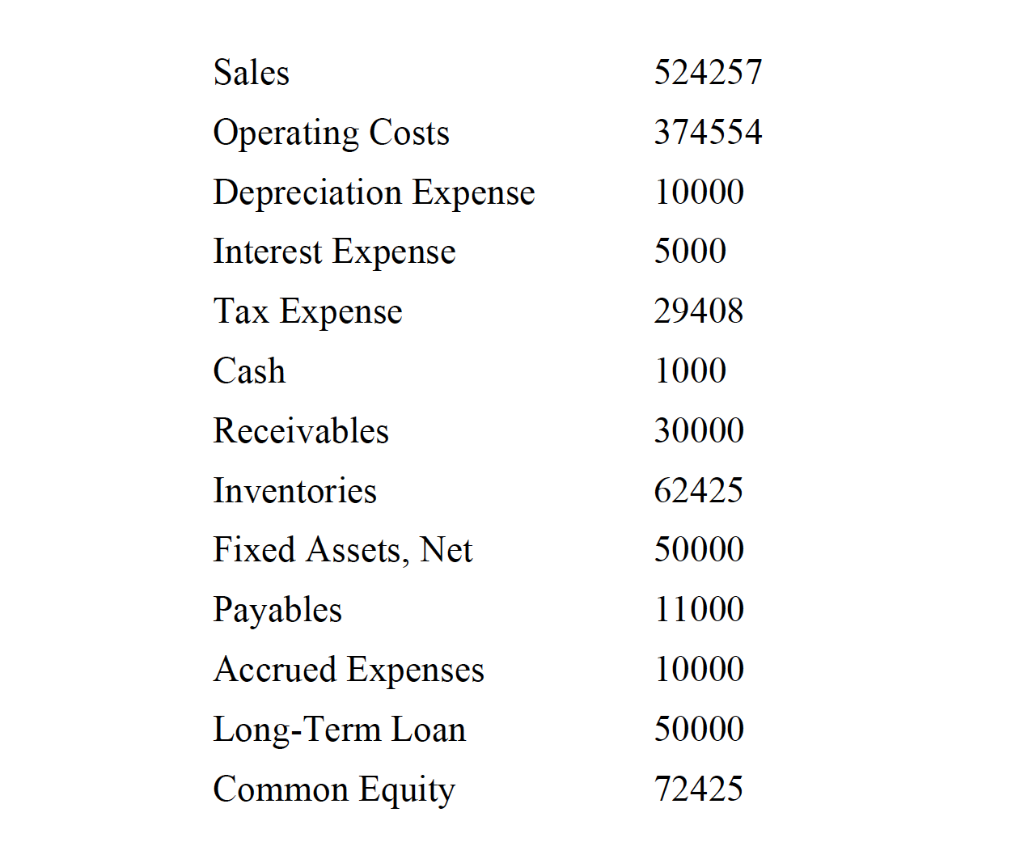524257 374554 10000 5000 29408 1000 Sales Operating Costs Depreciation Expense Interest Expense Tax Expense Cash Receivables Inventories Fixed Assets, Net Payables Accrued Expenses Long-Term Loan Common Equity 30000 62425 50000 11000 10000 50000 72425 Assignment: Prepare an income statement and a balance sheet for is company using the information provided. Calculate: 1. Current ratio 2. Quick ratio 3. NWC-to-total-Assets (Working capital to assets) 4. Ratio of total debt and liabilities to total assets 5. Ratio of total debt and...

• ### LongGone Corp. had total operating expenses of \$84 million last year, including depreciation, and paid \$5.2...

LongGone Corp. had total operating expenses of \$84 million last year, including depreciation, and paid \$5.2 million in interest. The company also paid out \$43 million in dividends, while the addition to retained earnings increased equity by 90%. The average tax rate was 34% and the average interest rate on the company's debt was 5%. The payout ratio was 30%. a) What was equity at the end of the year, before the addition of retained earnings (in \$ million)? b)...

• ### 511770 367062 10000 5000 29408 1000 Sales Operating Costs Depreciation Expense Interest Expense Tax Expense Cash...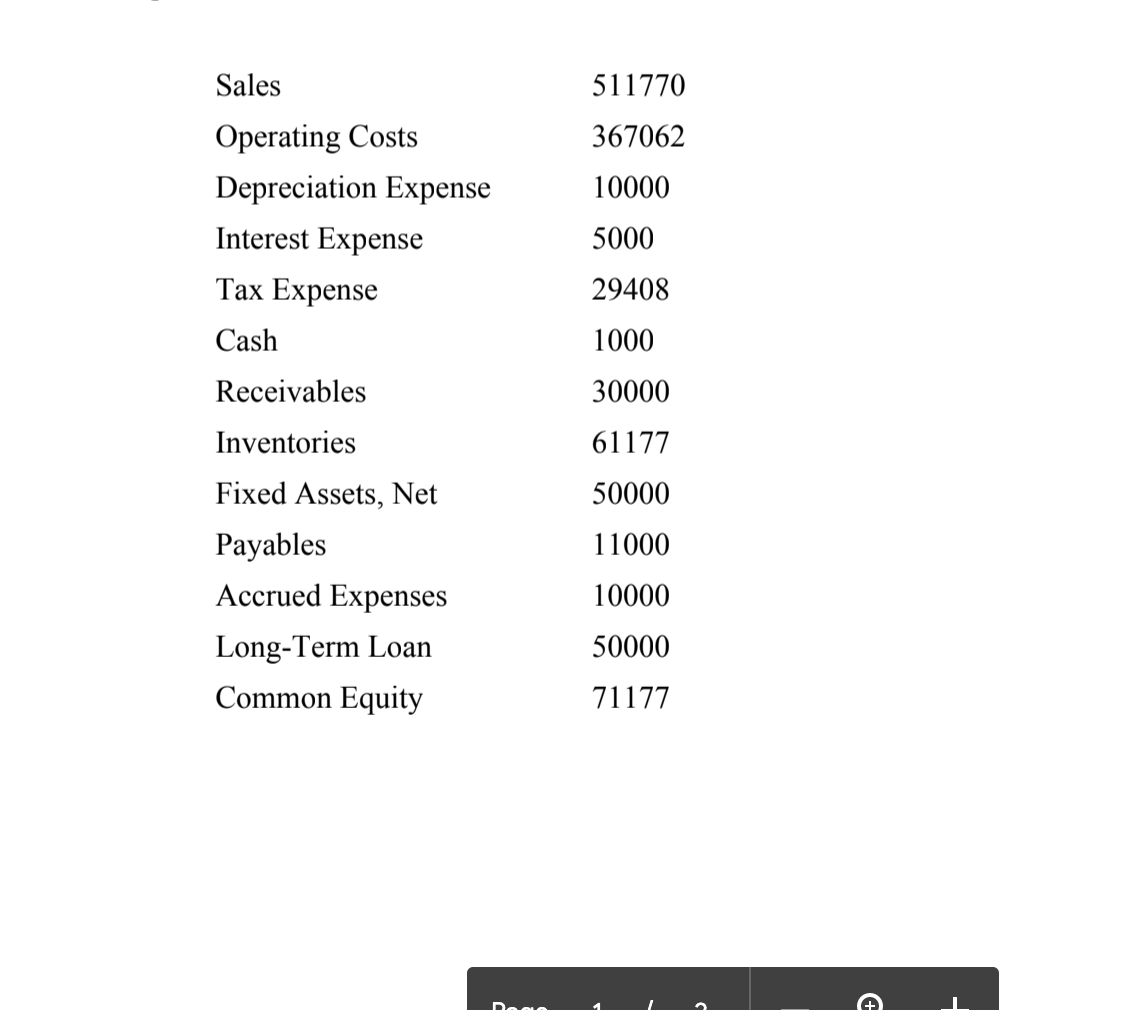511770 367062 10000 5000 29408 1000 Sales Operating Costs Depreciation Expense Interest Expense Tax Expense Cash Receivables Inventories Fixed Assets, Net Payables Accrued Expenses Long-Term Loan Common Equity 30000 61177 50000 11000 10000 50000 71177 1. Current ratio 2. Quick ratio 3. NWC-to-total-Assets (Working capital to assets) 4. Ratio of total debt and liabilities to total assets 5. Ratio of total debt and liabilities to shareholder's equity 6. Interest coverage 7. Net profit margin 8. Sales to total assets (Asset...

• ### 484283 350570 10000 5000 29408 1000 Sales Operating Costs Depreciation Expense Interest Expense Tax Expense Cash...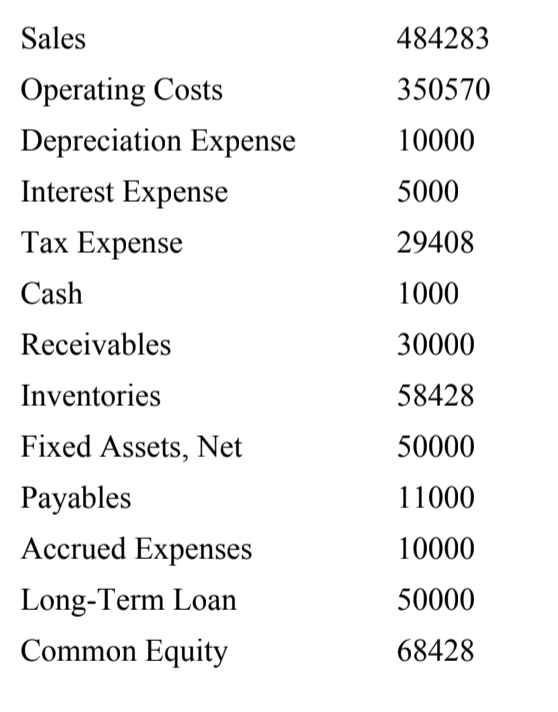484283 350570 10000 5000 29408 1000 Sales Operating Costs Depreciation Expense Interest Expense Tax Expense Cash Receivables Inventories Fixed Assets, Net Payables Accrued Expenses Long-Term Loan Common Equity 30000 58428 50000 11000 10000 50000 68428 1. Current ratio 2. Quick ratio 3. NWC-to-total-Assets (Working capital to assets) 4. Ratio of total debt and liabilities to total assets 5. Ratio of total debt and liabilities to shareholder's equity 6. Interest coverage 7. Net profit margin 8. Sales to total assets (Asset...

• ### Intro You've collected the following information about a company Value Line item Sales Costs Depreciation Interest...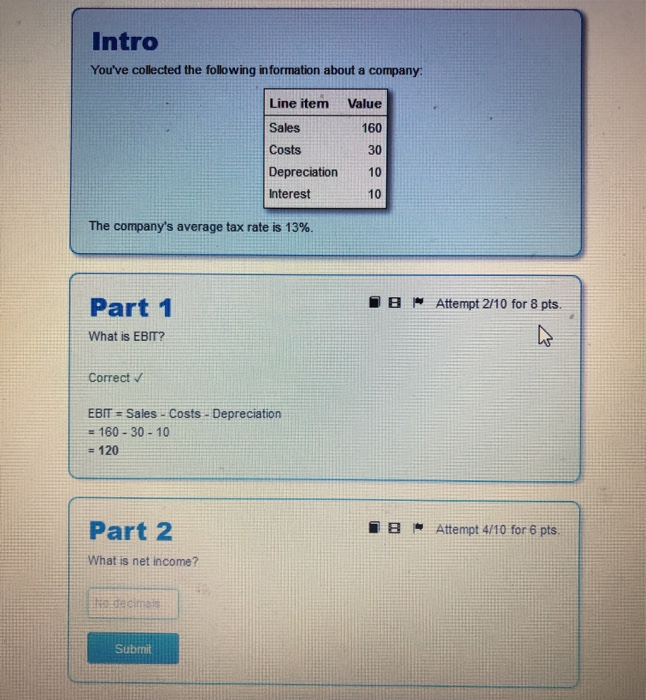Intro You've collected the following information about a company Value Line item Sales Costs Depreciation Interest The company's average tax rate is 13%. B Attempt 2/10 for 8 pts. Part 1 What is EBIT? Correct v EBIT = Sales - Costs - Depreciation = 160 - 30 - 10 = 120 Part 2 IS- Attempt 4/10 for 6 pts. What is net income? tecime Submit Intro You've collected the folowing information about a company Value 660 Line item Sales Costs...

• ### ILILIULUI JUNI,UJU IIT 2017. It also hou expenses (excluding depreciation) \$366,240, depreciation of \$103,471, and interest...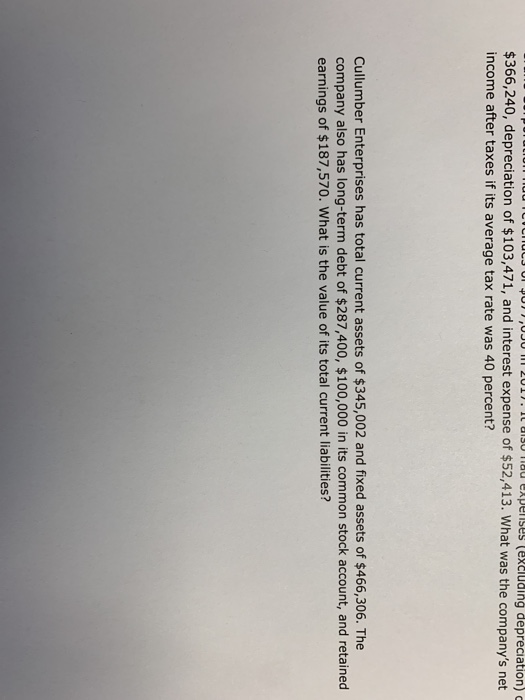ILILIULUI JUNI,UJU IIT 2017. It also hou expenses (excluding depreciation) \$366,240, depreciation of \$103,471, and interest expense of \$52,413. What was the company's net income after taxes if its average tax rate was 40 percent? Cullumber Enterprises has total current assets of \$345,002 and fixed assets of \$466,306. The company also has long-term debt of \$287,400, \$100,000 in its common stock account, and retained earnings of \$187,570. What is the value of its total current liabilities?

• ### 524257 374554 10000 5000 29408 1000 Sales Operating Costs Depreciation Expense Interest Expense Tax Expense Cash...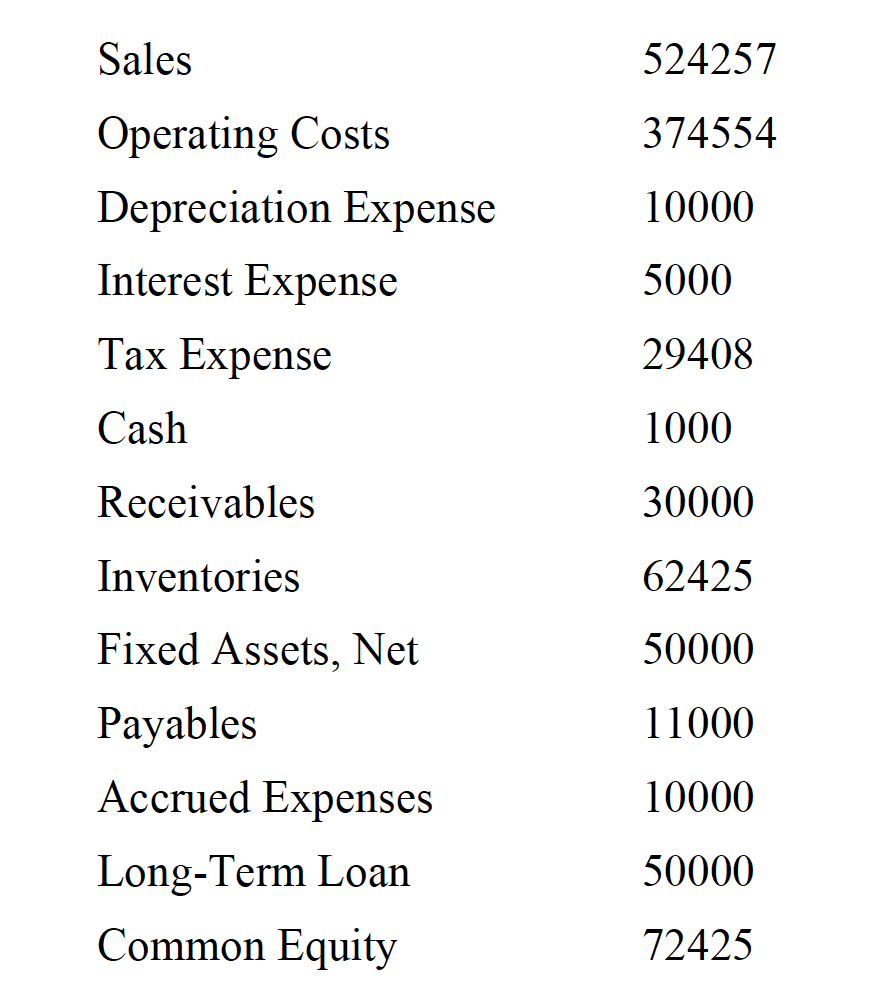524257 374554 10000 5000 29408 1000 Sales Operating Costs Depreciation Expense Interest Expense Tax Expense Cash Receivables Inventories Fixed Assets, Net Payables Accrued Expenses Long-Term Loan Common Equity 30000 62425 50000 11000 10000 50000 72425 5. Ratio of total debt and liabilities to shareholder's equity 6. Interest coverage 7. Net profit margin 8. Sales to total assets (Asset turnover) 9. Return on assets 10. Equity multiplier 11. Return on equity

• ### Intro LongGone Corp. had total operating expenses of \$84 million last year, including depreciation, and paid...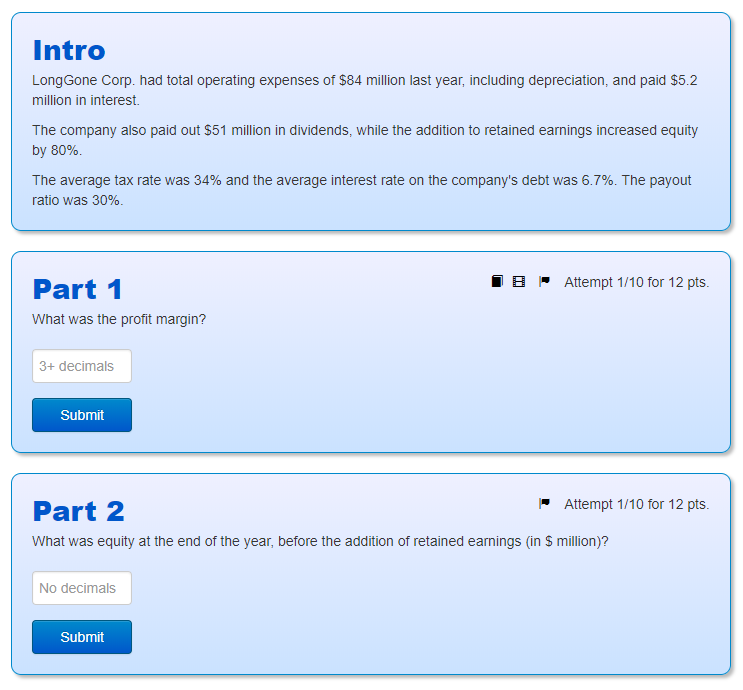Intro LongGone Corp. had total operating expenses of \$84 million last year, including depreciation, and paid \$5.2 million in interest. The company also paid out \$51 million in dividends, while the addition to retained earnings increased equity by 80%. The average tax rate was 34% and the average interest rate on the company's debt was 6.7%. The payout ratio was 30%. Part 1 What was the profit margin? E Attempt 1/10 for 12 pts. 3+ decimals Submit Attempt 1/10 for...